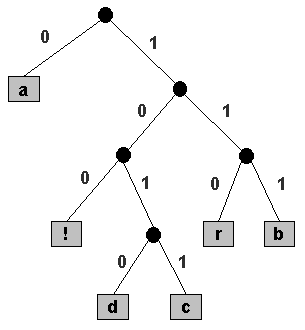COS 126 Data Compression Programming Assignment Due: Wednesday, 11:59pm

Write a program to decipher messages encoded using a prefix code, given the encoding tree. Such codes are widely used in applications that compress data, including JPEG for images, MP3 for music, and DivX for video.

Prefix codes.  A prefix code is most easily represented by a binary tree in which the external nodes are labeled with single characters that are combined to form the message. The encoding for a character is determined by following the path down from the root of the tree to the external node that holds that character: a 0 bit identifies a left branch in the path, and a 1 bit identifies a right branch. In the following tree, black circles are internal nodes and gray squares are external nodes. The code for `b` is `111`, because the external node holding `b` is reached from the root by taking 3 consecutive right branches. The other codes are given in the table below.```character encoding ------------------- a 0 b 111 c 1011 d 1010 r 110 ! 100 ```

Note that each character can be encoded with a different number of bits. In the example above, the character 'a' is encoded with a single bit, while the character 'd' is encoded with 4 bits. This is a fundamental property of prefix codes. In order for this encoding scheme to reduce the number of bits in a message, we use short encodings for frequently used characters, and long encodings for infrequent ones. A second fundamental property of prefix codes is that messages can be formed by simply stringing together the code bits from left to right. For example, the bitstring

`0111110010110101001111100100`

encodes the message "`abracadabra!`". The first 0 must encode `'a'`, then the next three 1's must encode `'b'`, then `110` must encode `r`, and so on as follows:

```|0|111|110|0|1011|0|1010|0|111|110|0|100
a  b   r  a  c   a  d   a  b   r  a  !
```

The codes can be run together because no encoding is a prefix of another one. This property defines a prefix code, and it allows us to represent the character encodings with a binary tree, as shown above. To decode a given bit string:

• Start at the root of the tree.
• Repeat until you reach an external leaf node.
• Read one message bit.
• Take the left branch in the tree if the bit is 0; take the right branch if it is 1.
• Print the character in that external node.
This whole process is repeated, starting over at the root, until all of the bits in the compressed message are exhausted. Your main task is to parse the binary tree and implement this procedure.

Representing the binary tree.  To decode a bit string, you need the binary tree that stores the character encodings. We use the preorder traversal of the binary tree to represent the tree itself. Internal nodes are labeled with the special character '*'. (For convenience, we artificially restrict ourselves to messages that do not contain this special character.) The preorder traversal of the above tree is:

`* a * * ! * d c * r b `

Input format.  The input will consist of the preorder traversal of the binary tree, followed immediately on a new line by the compressed message. For the example above, the input file is `abra.pre`:

```*a**!*dc*rb
0111110010110101001111100100
```
In order to read the input one character at a time, use the library CharStdIn.java.

Part 1: Building the tree.  Design a class PrefixTree to represent prefix trees. A PrefixTree should store a character (either an input symbol or the special character '*') and references to two subtrees. The beginning of your class might look like:

```public class PrefixTree {
private char character;
private PrefixTree left;
private PrefixTree right;
```
Design the constructor so that it reads in the preorder traversal of a tree from standard input, and reconstructs it.

Part 2: Tree traversal.  Include a method preorder that traverses the binary tree in preorder, and prints a list of characters in the tree, the length (number of bits) of their encoding, and the encoding. For the example above, your program should produce the following output.

```character  bits  encoding
-------------------------
a        1     0
!        3     100
d        4     1010
c        4     1011
r        3     110
b        3     111
```

Part 3: Uncompressing.  Now, write a method uncompress that reads the compressed message from standard input, and writes the uncompressed message to standard output. It should also display the number of bits read in, the number of characters in the original message, and the compression factor.

For example, the original message above contains 12 characters which would normally requires 96 bits of storage (8 bits per character). The compressed message uses only 28 bits, or 29% of the space required without compression. The compression factor depends on the frequency of characters in the message, but ratios around 50% are common for English text. Note that for large messages the amount of space needed to store the description of the tree is negligible compared to storing the message itself, so we have ignored this quantity in the calculation. Also, for simplicity, the compressed message is a sequence of the characters `'0'` and `'1'`. In an actual application, these bits would be packed eight to the byte, thus using 1/8th the space. For this input, your program should produce the following output:

```character  bits  encoding
-------------------------
a        1     0
!        3     100
d        4     1010
c        4     1011
r        3     110
b        3     111

abracadabra!
Number of bits       = 28
Number of characters = 12
Compression ratio    = 29.166666666666668%
```
Testing and debugging.  You can test your program on the inputs in the directory `/u/cs126/files/prefix/`.

Submission.  Name your program PrefixTree.java, and submit it along with a readme.txt file.

This assignment was created by Robert Sedgewick and Kevin Wayne.
Copyright © 2000 Robert Sedgewick